Welcome To Basics In Maths

# Addition of Vectors (Qns.& Ans) V.S.A.Q.’S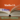# These solutions designed by the ‘Basics in Maths’ team. These notes to do help the intermediate First-year Maths students.

### Inter Maths – 1A two marks questions and solutions are very useful in IPE examinations.

#### QUESTION 1

Find the unit vector in the direction of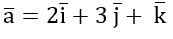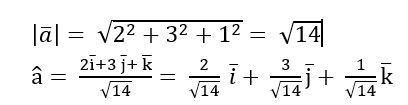#### QUESTION 2

Find a vector in the direction of a where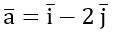that has a magnitude of 7 units.

The vector having the magnitude 7 and in the direction of  is

#### QUESTION 3

Find the unit vector in the direction of the sum of the vectors, a = 2i + 2j – 5k and b = 2i + j + 3k

Sol Given vectors are a = 2i + 2j – 5k and b = 2i + j + 3k

a + b = (2i + 2j – 5k) + (2i + j + 3k) = 4i + 3j – 2k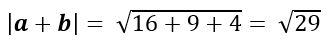##### QUESTION 4

Write the direction cosines of the vector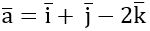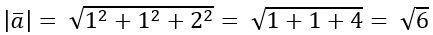QUESTION 5

Show that the points whose position vectors are – 2a + 3b + 5c, a + 2b + 3c, 7 ac are collinear when a, b, c are non-collinear vectors

Sol: Let OA = – 2a + 3b + 5c, OB = a + 2b + 3c, OC = 7 acA

B = OB – OA = a + 2b + 3c (– 2a + 3b + 5c)

AB = 3a b – 2c

AC = OC – OA = 7 ac(– 2a + 3b + 5c)

AC = 9a – 3b – 6c = 3(3a b – 2c)

AC = 3 AB

A, B and C are collinear

##### QUESTION 6

ABCD is a parallelogram if L and M are middle points of BC and CD. Then find (i) AL and AM in terms of AB and AD (ii) 𝛌, if AM = 𝛌 AD – LM

Sol: Given, ABCD is a parallelogram and L and M are middle points of BC and CD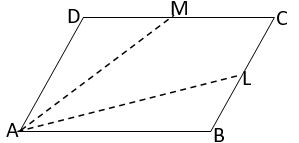(i) Take A as the origin

M is the midpoint of CD

= AD + ½ AB (∵ AB = DC)

L is the midpoint of BC

(ii) AM = 𝛌 AD – LM

𝛌 = 3/2

#### QUESTION 7

If G is the centroid of the triangle ABC, then show that OG =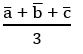when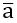,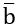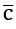are the position vectors of the vertices of triangle ABC.

Sol: OA = a, OB = b, OC = c and OD = d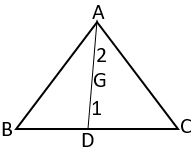D is the midpoint of BC

G divides median AD in the ratio 2: 1

##### QUESTION 8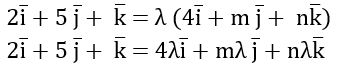Equating like vectors

2 = 4 λ; 5 = m λ; 1 = n λ

∴ m = 10, n = 2

#### QUESTION 9

The unit vector in the direction of a + b =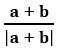##### QUESTION 10

If the vectors – 3i + 4j + λk and μi + 8j + 6k. are collinear vectors, then find λ and μ.

Sol: let a = – 3i + 4j + λk, b = μi + 8j + 6k

⟹   a = tb

– 3i + 4j + λk = t (μi + 8j + 6k)

– 3i + 4j + λk = μt i + 8t j + 6t k

Equating like vectors

– 3 = μt; 4 = 8t, λ = 6t

4 = 8t

∴ μ=– 6, λ = 3

##### QUESTION 11

ABCD is a pentagon. If the sum of the vectors AB, AE, BC, DC, ED and AC is 𝛌 AC then find the value of 𝛌

Sol: Given, ABCD is a pentagon

AB + AE + BC + + DC + ED + AC = 𝛌 AC

(AB + BC) + (AE + DC + ED) + AC = 𝛌 AC

AC + AC + AC = 𝛌 AC

3 AC = 𝛌 AC

𝛌 = 3

#### QUESTION 12

If the position vectors of the points A, B and C are – 2i + jk and –4i + 2j + 2k and 6i – 3j – 13k respectively and AB = 𝛌 AC, then find the value of 𝛌

Sol: Given, OA = – 2i + jk , OB = –4i + 2j + 2k and OC  = 6i – 3j – 13k

AB = OB – OA = –4i + 2j + 2k – (– 2i + jk)

= –4i + 2j + 2k +2ij + k

= –2i + j + 3k

AC = OC – OA = 6i – 3j – 13k – (– 2i + jk)

= 6i – 3j – 13k +2ij + k

= 8i –4 j –12k

= – 4 (2i + j + 3k)

AC = – 4 AB

Given AB = 𝛌 AC

𝛌 = – 1/4

#### QUESTION 13

If OA = i + j +k, AB = 3i – 2j + k, BC = i + 2j – 2k, CD = 2i + j +3k then find the vector OD

Sol: Given OA = i + j +k, AB = 3i – 2j + k, BC = i + 2j – 2k, CD = 2i + j +3k

OD = OA + AB + BC + CD

= i + j +k + 3i – 2j + k + i + 2j – 2k + 2i + j +3k

= 7i + 2j +4k

#### QUESTION 14

Let a = 2i +4 j –5 k, b = i + j+ k, c = j +2 k, then find the unit vector in the opposite direction of a + b + c

Sol:  Given, a = 2i +4 j –5 k, b = i + j+ k, c = j +2 k

a + b + c = 2i +4 j –5 k + i + j+ k + j +2 k

= 3i +6j –2k

The unit vector in the opposite direction of a + b + c is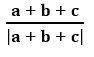##### QUESTION 15

Is the triangle formed by the vectors 3i +5j +2k, 2i –3j –5k, 5i – 2j +3k

Sol: Let a =3i +5j +2k, b = 2i –3j –5k, c = 5i – 2j +3k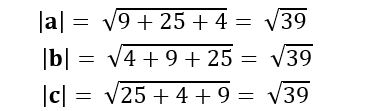∴ Given vectors form an equilateral triangle.

##### QUESTION 16

Using the vector equation of the straight line passing through two points, prove that the points whose position vectors are a, b,  (3a – 2b) are collinear.

Sol: the vector equation of the straight line passing through two points a, b is

r = (1 – t) a+ t b

3a – 2b = (1 – t) a+ t b

Equating like vectors

1 – t = 3 and t = – 2

∴ Given points are collinear.

###### QUESTION 17

OABC is a parallelogram If OA = a and OC = c, then find the vector equation of the side BC

Sol: Given, OABC is a parallelogram and OA = a, OC = c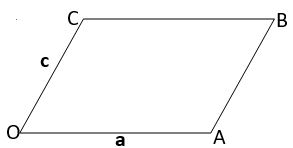The vector equation of BC is a line which is passing through C(c) and parallel to OA

⟹ the vector equation of BC is r = c + t a

##### QUESTION 18

If a, b, c are the position vectors of the vertices A, B, and C respectively of triangle ABC, then find the vector equation of the median through the vertex A

Sol: Given OA = a, OB = b, OC = c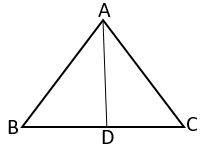D is mid of BC

#### QUESTION 19

Find the vector equation of the line passing through the point 2i +3j +k and parallel to the vector 4i – 2j + 3k

Sol: Let a =2i +3j +k, b = 4i – 2j + 3k

The vector equation of the line passing through a and parallel to the vector b is r = a + tb

r = 2i +3j +k + t (4i – 2j + 3k)

= (2 + 4t) i + (3 – 2t) j + (1 + 3t) k

##### QUESTION 20

Find the vector equation of the plane passing through the points i – 2j + 5k, 2j –k and – 3i + 5j

Sol: The vector equation of the line passing through a, b and cis r = (1 – t – s) a + tb + sc

r = (1 – t – s) (i – 2j + 5k) + t (2j –k) + s (– 3i + 5j)

= (1 – t – 4s) i + (– 2 – 3t + 7s) j + (5 – 6t – 5s) k

####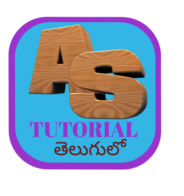error: Content is protected !!